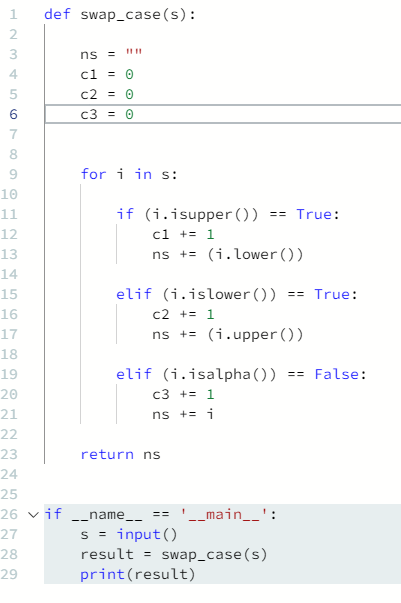# Hackerrank – Python – sWAP cASE – Solution

Publish Date - February 8th, 2023

|

This solution was fun to solve. It has it all, loops, conditionals, string manipulation, variables and functions. While you may be able to use the function swapcase(), I think it’s a great way to show Jr. Python developers how to mix everything together! While it was harder than the Tuple’s solution and easier than runner up score it doesn’t hold a light at all to some of the MySQL challenges in terms of complexity. Let’s jump into the problem and solution as always:

## The problem

You are given a string and your task is to swap cases. In other words, convert all lowercase letters to uppercase letters and vice versa.

For Example:

``````Www.HackerRank.com → wWW.hACKERrANK.COM
Pythonist 2 → pYTHONIST 2
``````

Function Description

Complete the swap_case function in the editor below.

swap_case has the following parameters:

• string s: the string to modify

Returns

• string: the modified string

Input Format

A single line containing a string .

Constraints

0 <= len(s) <= 1000

Sample Input 0

```HackerRank.com presents "Pythonist 2".
```

Sample Output 0 (Is your desired output).

`hACKERrANK.COM PRESENTS "pYTHONIST 2".`

## The solution

``````def swap_case(s):

ns = ""
c1 = 0
c2 = 0
c3 = 0

for i in s:

if (i.isupper()) == True:
c1 += 1
ns += (i.lower())

elif (i.islower()) == True:
c2 += 1
ns += (i.upper())

elif (i.isalpha()) == False:
c3 += 1
ns += i

return ns

if __name__ == '__main__':
s = input()
result = swap_case(s)
print(result)``````

There’s multiple layers for my solution, so I’ll break it down and summarize everything together.

If I were to write this out from a logic standpoint it would be like this:

There’s a function (swap_case) that does the following:

• set an empty “new string” with three other variables as generic counters (c1, c2, c3)
• loop through “s” individual with “i” (so each letter of “s” which is HackerRank.com presents “Pythonist 2”.)
• and then each IF clause:
• if “i” (which is a letter, number or non-alpha) is uppercase, count each one and assign each uppercase letter as a lower case letter to the new string.
• if “i” (which is a letter, number or non-alpha) is lowercase, count each one and assign each lowercase letter as a lower uppercase to the new string.
• if “i” (which is a letter, number or non-alpha) is not an alphanumeric, count each one and assign it to the new string. Note: that I didn’t change the non-alphanumeric).
• Next, I return “ns” (new string) so that it can be passed in the following “result”, when the user input’s something (s).

``hACKERrANK.COM PRESENTS "pYTHONIST 2".``

Some custom input and outputs:

Input: tHERE wAS aCOOL pERSON tHAT wAS vERY sMART

Output: There Was Acool Person That Was Very Smart

Input: mACHINE lEARNING iS vERY cOOL

Output: Machine Learning Is Very Cool

Input: i LoVe NaTuRaL LaNgUaGe PrOcEsSiNg

Output: I lOvE nAtUrAl lAnGuAgE pRoCeSsInG

## Conclusion

Like I said, this is one of my favorite Python examples to date. It gives you a little bit of everything and actually has some genuine use cases. You could use this as a script to title case all the content on a webpage or title-tags across a website. Web development concepts aside, there are plenty of other applications to shape a function like this. I hope this article helped you solve a fun question!